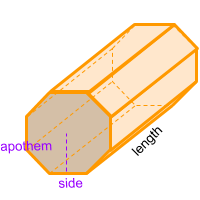Home » Polyhedra » Prisms » Octagonal Prism

# Volume of an Octagonal PrismVolume =
4 × Side × Apothem
× Length

## Volume calculator for an octagonal prism

Enter a Side and the Apothem of the octagon that forms one of the bases of the octagonal prism along the length of the prism to get the volume automatically.

If you don't know the value of the apothem you can enter a side of the octagon that forms one of the bases of the octagonal prism along the length of the prism to get the value automatically.

## Description, how many faces, edges and vertices are there in an octagonal prism

The octagonal prism, is a figure formed by 10 faces, 2 of which are equal and parallel octagons, and form the bases at the ends of the shape. Another 8 faces are parallelograms. It has 24 edges and 16 vertices. If a cross section is made in any part of its length, it always maintains the shape of an octagon.

## Formula for the volume of an octagonal prism

To calculate the volume of an octagonal prism you can use the formula to get the volume of all prisms, where the area of the base of the prism is multiplied by the length of the prism. In this case, the base of the octagonal prism is an octagon, therefore, the area of the octagon need to be a known value.

To calculate the area of the octagon (base area), multiply the perimeter of the octagon by its apothem and divide by two. Then to get the volume of the octagonal prism you need to multiply the result by the length of the prism.

Remember that the apothem is the distance from the center of the polygon to the middle of one of its sides. And also remember that the perimeter of an octagon is the sum of all its sides. You can also use the online calculator to calculate the volume of the octagonal prism automatically.

Volume =
4 × Side × Apothem
× Length

Formula explanation and alternative formula:

The formula to calculate the volume of prism is always the same:

Volume prism = Area base × Length

In this case, the area of the base of the octagonal prism is a octagon:

Area base = Area octagon =
Perimeter × Apothem/2

To calculate the perimeter:

Perimeter = 8 × Side

Replacing the perimeter formula in the formula shown above, then you get the main formula.

To get the volume of an octagonal prism without knowing the apothem:

Apothem =
side/2•tan(α÷2)
, with
α =
360°/8

Therefor, another way to get the volume of an octagonal prism without knowing the apothem is:

Volume =
2 × side ² / Tan(22,5°)
× length

Share